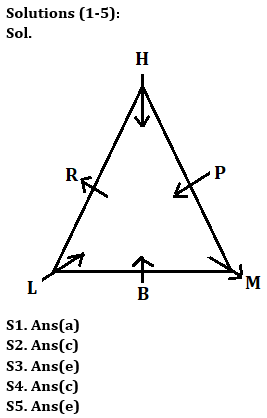Latest Banking jobs   »

# Reasoning Quiz For ECGC PO 2023 -28th April

Directions (1-5): Study the information carefully and answer the questions given below.
Six persons are sitting around a triangular table. Some of them face towards the center and some of them face outside the center. Three persons sits each of the corner of table and rest sits middle of each side of table.
P sits second to the right of B. One person sits between H and M. R is not immediate neighbor of P. L and R sits immediate left to each other. R does not sit at corner of triangle. P face towards center and M does not sit immediate right of P. More than three persons face towards center. M and L face opposite direction to each other. M does not face towards center. R is not immediate neighbor of B. L is not an immediate neighbour of P.

Q1. Who among the following sits immediate right of R?
(a) H
(b) L
(c) P
(d) B
(e) None of these

Q2. Which of the following group of persons sit at corner of table?
(a) H, P, M
(b) H, B, P
(c) M, L, H
(d) H, R, L
(e) None of these

Q3. Who among the following sits third to the right of L?
(a) M
(b) H
(c) R
(d) B
(e) None of these

Q4. Which of the following statement is true about P?
(a) P sits immediate right of M
(b) Two persons sit between P and R
(c) No one sit between H and P
(d) P sits fourth to the right of B
(e) None is true

Q5. How many persons face outside the center?
(a) One
(b) Three
(c) Four
(d) None
(e) Two

Directions (6-10): Answer the following questions referring to the symbol-letter-number sequence given below:

P G 5 O S 2 # A & L N 1 M 3 G B R 9 D 6 Y 4 % J 7 E

Q6. Which of the following element is the third to the right of fourteenth element from the left end?
(a) R
(b) N
(c) 7
(d) %
(e) None of these

Q7. How many letters are there in the above sequence which are immediately preceded by a number and immediately followed by a consonant?
(a) None
(b) One
(c) Two
(d) Three
(e) None of these

Q8. What should come in place of the question mark (?) in the following sequence?
?, 2LO, &M#
(a) 5#K
(b) OAB
(c) SA5
(d) 5#M
(e) None of these

Q9. Which of the following is the eleventh element to the right of the second element from the left end in the above sequence?
(a) 9
(b) 1
(c) Y
(d) 4
(e) None of these

Q10. If first 13 element of the series are written in reverse order, then which of the following element is 8th to the left of the element which is 10th from the right end in the new arrangement?
(a) L
(b) S
(c) 2
(d) O
(e) None of these

Directions (11-15): In the following questions, the symbols @, #, %, \$ and © are used with the following meaning as illustrated below-
‘P#Q’ means ‘P is neither greater than nor equal to Q’
‘P*Q’ means ‘P is neither equal to nor smaller than Q’
‘P%Q’ means ‘P is neither smaller than nor greater than Q’
‘P\$Q’ means ‘P is not smaller than Q’
‘P@Q’ means ‘P is not greater than Q’
Now in each of the following questions assuming the given statement to be true, find which of the three conclusions I, II and III given below them is/are definitely true and give your answer accordingly.

Q11. Statements: A@T#Y\$U%O*P@L\$M
Conclusions:
I. O*A
II. A@O
III. Y\$P
(a) None is true
(b) Only I is true
(c) Only III is true
(d) Either I or II are true
(e) None of these

Q12. Statements: Q%W\$Y%U@Z*N#M
Conclusions:
I. Z*Y
II. N#W
III. Y%Z
(a) Only I and III are true
(b) Only II and III are true
(c) Only I and II are true
(d) All are true
(e) None of these

Q13. Statements: R*E#A@C%T\$K@R%O
Conclusions:
I. T*E
II. K@C
III. R%A
(a) None is true
(b) Only II is true
(c) Only I and II are true
(d) Only II and III are true
(e) All are true

Q14. Statements: V@B%R*E#I%M\$X
Conclusions:
I. R*I
II. B*E
III. E#M
(a) None is true
(b) Only II is true
(c) Only I and II are true
(d) Only II and III are true
(e) All are true

Q15. Statements: X#Y@U*Z\$H%R*E\$T
Conclusions:
I. T@U
II. U*T
III. Y*H
(a) None is true
(b) Only II and III are true
(c) Either I and II are true
(d) Only II is true
(e) All are true

SolutionsSolutions (6-10):
S6. Ans(a)
S7. Ans(c)
Sol. 5OS, 3GB
S8. Ans(e)
S9. Ans(e)
S10.Ans(b)

Solutions (11-15):
S11. Ans(a)
S12. Ans(e)
S13. Ans(c)
S14. Ans(d)
S15. Ans(d)## FAQs

### What is the total duration of ECGC PO for objective?

The duration of ECGC PO for objective is 140 minutes

#### Congratulations!Union Budget 2023-24: Free PDF